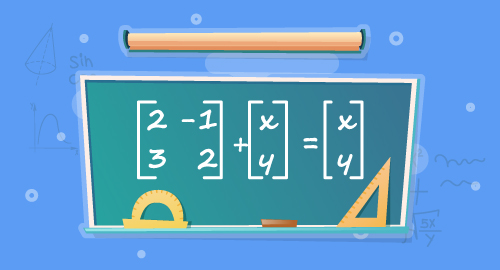#### Need Help?

Get in touch with usSAT/ACT

# System of Linear Equations

Maths

Solve system of linear equation by inspection. Understand the solution to the system of linear equation by using graphs. Solve the system of two linear equations in two variables algebraically.

##### Course Duration
•2 Weeks4 Sessions
•50 mins per session
##### Tutor## What you'll learn

• 1

Find the number of solutions of a system of equations by inspecting the equations.

• 2

Find the solution to the system of equation by using graphs.

• 3

Solve system of equations using substitution and elimination method.

## Skills you'll learnProblem solvingInfering graphs